##### Differential Equations For Dummies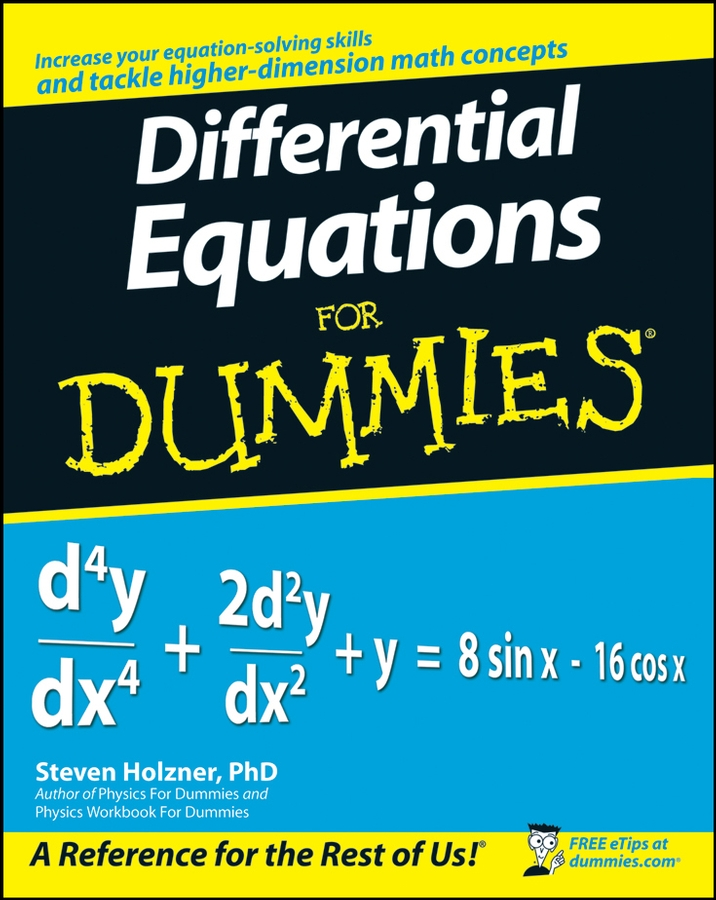A line is the simplest function that you can graph on the coordinate plane. (Lines are important in calculus because when you zoom in far enough on a curve, it looks and behaves like a line.) This figure shows the graph of the line y = 3x + 5.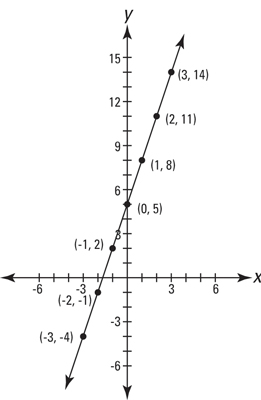## Hitting the slopes

The most important thing about the line in this figure — at least, for your study of calculus — is its slope or steepness. Notice that whenever x goes 1 to the right, y goes up by 3. A good way to visualize slope is to draw a stairway under the line (see the figure, below).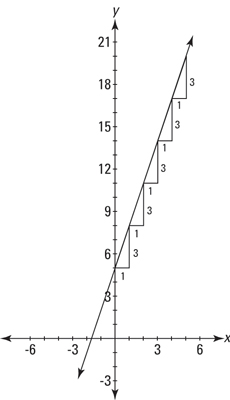The vertical part of the step is called the rise, the horizontal part is called the run, and the slope is defined as the ratio of the rise to the run.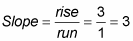(As you’ll see below, you don’t have to make the run equal to 1 to calculate the slope of a line, but if you make the run equal to 1, the slope is the same as the rise because a number divided by 1 equals itself. This is a good way to think about slope — the slope is the amount that a line goes up (or down) as it goes 1 to the right.)

Lines that go up to the right have a positive slope; lines that go down to the right have a negative slope. Horizontal lines have a slope of zero, and vertical lines don't have a slope — you say that the slope of a vertical line is undefined.

It’s easy to see that the slope of the above line is 3 (either from the rise and run of the steps drawn on the second figure, or from coordinates on the first figure like (1, 8) and (2, 11) where you go over 1 and up 3). But in many problems, it won’t be that obvious, so you’ll need to use the slope formula. Here it is: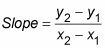Pick any two points on the line in the first figure, say (1, 8) and (3, 14), and plug them into the formula to calculate the slope: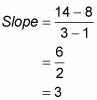Note that it doesn’t matter if you reverse the order of the points in the formula: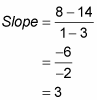Keep in mind that if you reverse only the coordinates in the numerator or only the ones in the denominator, you’ll get the wrong answer.

## Graphing lines

If you have the equation of the line y = 3x + 5, but not its graph, you can graph the line the old-fashioned way or with your graphing calculator:

• The old-fashioned way: Create a table of values by plugging numbers into x and calculating y. If you plug 0 into x, y equals 5; plug 1 into x, and y equals 8; plug 2 into x, and y is 11, and so on. Plot the points, connect the dots, and put arrows on both ends — there’s your line. (Note: the more complicated the shape of the graph, the more points you’ll need to see where the graph goes…you know, where it goes up and down, where it changes direction, and so on. With a simple line like the one above, while you might sometimes want to compute three or four points, only two points are needed for graphing the line.)

• With a graphing calculator: Just enter y = 3x + 5 and your calculator graphs the line and produces a table of x- and y-coordinates.

## Slope-intercept form

You can see that the line in the figure crosses the y-axis at 5 — this point is the y-intercept of the line. Because both the slope of 3 and the y-intercept of 5 appear in the equation y = 3x + 5, this equation is said to be in slope-intercept form. Here’s the form written in the general way:
y = mx + b. (Where m is the slope and b is the y-intercept.)

All lines, except for vertical lines, can be written in this form. Vertical lines always look like x = 6. The number tells you where the vertical line crosses the x-axis.

The equation of a horizontal line looks different — y = 10, for example. But it technically fits the form y = mx + b — it’s just that because the slope of a horizontal line is zero, and because zero times x is zero, there is no x-term in the equation.

A line is the simplest type of function, and a horizontal line (called a constant function) is the simplest type of line. It’s nonetheless fairly important in calculus, so make sure you know that a horizontal line has an equation like y = 10 and that its slope is zero.

If m = 1 and b = 0, you get the function y = x. This line goes through the origin (0, 0) and makes a 45-degree angle with both coordinate axes. It’s called the identity function because its outputs are the same as its inputs.

## Point-slope form

The point-slope form requires that you know — you guessed it — a point on a line and the line’s slope. You can use any point on the line. Here's the point-slope form: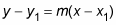Using the line in the preceding figure, pick any point — say (2, 11).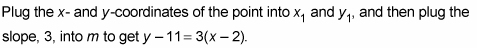With a little algebra, you can convert this equation into the one you already know: y = 3x + 5.